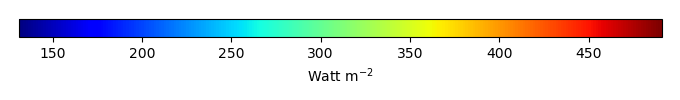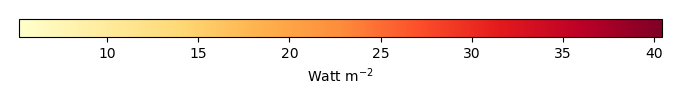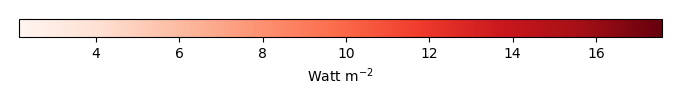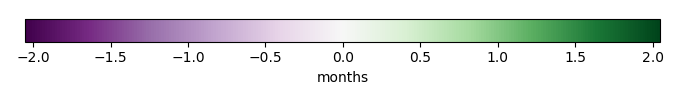# Mean State

Period Mean (original grids) [Watt m-2]
Model Period Mean (intersection) [Watt m-2]
Model Period Mean (complement) [Watt m-2]
Benchmark Period Mean (intersection) [Watt m-2]
Benchmark Period Mean (complement) [Watt m-2]
Bias [Watt m-2]
RMSE [Watt m-2]
Phase Shift [months]
Bias Score 
RMSE Score 
Seasonal Cycle Score 
Spatial Distribution Score 
Interannual Variability Score 
Overall Score 
Benchmark [-] 453.
CLM5PHSOFF [-] 462. 462. 0.00 453. 460. 9.50 15.9 1.55 0.36 0.37 0.78 0.99 0.73 0.60
CLM5PHSON [-] 462. 462. 0.00 453. 460. 9.34 15.7 1.72 0.34 0.37 0.75 0.99 0.71 0.59
Period Mean (original grids) [Watt m-2]
Model Period Mean (intersection) [Watt m-2]
Model Period Mean (complement) [Watt m-2]
Benchmark Period Mean (intersection) [Watt m-2]
Benchmark Period Mean (complement) [Watt m-2]
Bias [Watt m-2]
RMSE [Watt m-2]
Phase Shift [months]
Bias Score 
RMSE Score 
Seasonal Cycle Score 
Spatial Distribution Score 
Interannual Variability Score 
Overall Score 
Benchmark [-] 471.
CLM5PHSOFF [-] 482. 482. 0.00 471. 456. 10.5 20.6 0.623 0.56 0.52 0.95 0.91 0.74 0.70
CLM5PHSON [-] 481. 482. 0.00 471. 456. 10.1 20.9 0.713 0.56 0.50 0.95 0.95 0.71 0.69
Period Mean (original grids) [Watt m-2]
Model Period Mean (intersection) [Watt m-2]
Model Period Mean (complement) [Watt m-2]
Benchmark Period Mean (intersection) [Watt m-2]
Benchmark Period Mean (complement) [Watt m-2]
Bias [Watt m-2]
RMSE [Watt m-2]
Phase Shift [months]
Bias Score 
RMSE Score 
Seasonal Cycle Score 
Spatial Distribution Score 
Interannual Variability Score 
Overall Score 
Benchmark [-] 443.
CLM5PHSOFF [-] 454. 454. 0.00 443. 444. 10.2 18.5 0.793 0.51 0.53 0.90 0.99 0.64 0.68
CLM5PHSON [-] 451. 451. 0.00 443. 444. 7.63 17.9 1.14 0.57 0.49 0.85 0.99 0.61 0.67
Period Mean (original grids) [Watt m-2]
Model Period Mean (intersection) [Watt m-2]
Model Period Mean (complement) [Watt m-2]
Benchmark Period Mean (intersection) [Watt m-2]
Benchmark Period Mean (complement) [Watt m-2]
Bias [Watt m-2]
RMSE [Watt m-2]
Phase Shift [months]
Bias Score 
RMSE Score 
Seasonal Cycle Score 
Spatial Distribution Score 
Interannual Variability Score 
Overall Score 
Benchmark [-] 276.
CLM5PHSOFF [-] 281. 278. 0.00 275. 292. 3.71 15.7 0.0619 0.90 0.80 1.0 0.99 0.79 0.88
CLM5PHSON [-] 275. 275. 0.00 275. 292. 3.64 16.0 0.0557 0.90 0.80 1.0 0.99 0.79 0.88
Period Mean (original grids) [Watt m-2]
Model Period Mean (intersection) [Watt m-2]
Model Period Mean (complement) [Watt m-2]
Benchmark Period Mean (intersection) [Watt m-2]
Benchmark Period Mean (complement) [Watt m-2]
Bias [Watt m-2]
RMSE [Watt m-2]
Phase Shift [months]
Bias Score 
RMSE Score 
Seasonal Cycle Score 
Spatial Distribution Score 
Interannual Variability Score 
Overall Score 
Benchmark [-] 456.
CLM5PHSOFF [-] 458. 458. 0.00 453. 465. 5.06 10.0 1.72 0.32 0.40 0.73 0.94 0.71 0.59
CLM5PHSON [-] 458. 458. 0.00 453. 465. 5.84 10.1 1.76 0.31 0.41 0.73 0.93 0.72 0.59
Period Mean (original grids) [Watt m-2]
Model Period Mean (intersection) [Watt m-2]
Model Period Mean (complement) [Watt m-2]
Benchmark Period Mean (intersection) [Watt m-2]
Benchmark Period Mean (complement) [Watt m-2]
Bias [Watt m-2]
RMSE [Watt m-2]
Phase Shift [months]
Bias Score 
RMSE Score 
Seasonal Cycle Score 
Spatial Distribution Score 
Interannual Variability Score 
Overall Score 
Benchmark [-] 306.
CLM5PHSOFF [-] 317. 317. 0.00 306. 329. 11.4 15.4 0.0176 0.84 0.87 1.0 0.99 0.89 0.91
CLM5PHSON [-] 316. 315. 0.00 306. 329. 10.1 14.8 0.0215 0.86 0.86 1.0 1.0 0.89 0.91
Period Mean (original grids) [Watt m-2]
Model Period Mean (intersection) [Watt m-2]
Model Period Mean (complement) [Watt m-2]
Benchmark Period Mean (intersection) [Watt m-2]
Benchmark Period Mean (complement) [Watt m-2]
Bias [Watt m-2]
RMSE [Watt m-2]
Phase Shift [months]
Bias Score 
RMSE Score 
Seasonal Cycle Score 
Spatial Distribution Score 
Interannual Variability Score 
Overall Score 
Benchmark [-] 362.
CLM5PHSOFF [-] 367. 366. 0.00 361. 395. 6.05 15.6 0.132 0.85 0.82 0.99 0.99 0.73 0.87
CLM5PHSON [-] 364. 363. 0.00 361. 395. 4.75 15.4 0.151 0.86 0.82 0.99 0.99 0.73 0.87
Period Mean (original grids) [Watt m-2]
Model Period Mean (intersection) [Watt m-2]
Model Period Mean (complement) [Watt m-2]
Benchmark Period Mean (intersection) [Watt m-2]
Benchmark Period Mean (complement) [Watt m-2]
Bias [Watt m-2]
RMSE [Watt m-2]
Phase Shift [months]
Bias Score 
RMSE Score 
Seasonal Cycle Score 
Spatial Distribution Score 
Interannual Variability Score 
Overall Score 
Benchmark [-] 450.
CLM5PHSOFF [-] 456. 456. 0.00 450. 463. 6.85 18.2 0.649 0.61 0.57 0.94 1.0 0.64 0.72
CLM5PHSON [-] 452. 452. 0.00 450. 463. 4.66 17.7 0.767 0.65 0.56 0.92 1.0 0.63 0.72
Period Mean (original grids) [Watt m-2]
Model Period Mean (intersection) [Watt m-2]
Model Period Mean (complement) [Watt m-2]
Benchmark Period Mean (intersection) [Watt m-2]
Benchmark Period Mean (complement) [Watt m-2]
Bias [Watt m-2]
RMSE [Watt m-2]
Phase Shift [months]
Bias Score 
RMSE Score 
Seasonal Cycle Score 
Spatial Distribution Score 
Interannual Variability Score 
Overall Score 
Benchmark [-] 275.
CLM5PHSOFF [-] 282. 283. 0.00 275. 280. 8.28 15.3 0.0348 0.87 0.82 1.0 0.99 0.85 0.89
CLM5PHSON [-] 282. 282. 0.00 275. 280. 7.91 15.3 0.0355 0.87 0.82 1.0 1.0 0.85 0.89
Period Mean (original grids) [Watt m-2]
Model Period Mean (intersection) [Watt m-2]
Model Period Mean (complement) [Watt m-2]
Benchmark Period Mean (intersection) [Watt m-2]
Benchmark Period Mean (complement) [Watt m-2]
Bias [Watt m-2]
RMSE [Watt m-2]
Phase Shift [months]
Bias Score 
RMSE Score 
Seasonal Cycle Score 
Spatial Distribution Score 
Interannual Variability Score 
Overall Score 
Benchmark [-] 448.
CLM5PHSOFF [-] 447. 448. 0.00 450. 423. -1.97 14.5 0.320 0.77 0.71 0.97 0.99 0.71 0.81
CLM5PHSON [-] 449. 450. 0.00 450. 423. -3.08 14.6 0.337 0.78 0.71 0.97 0.99 0.70 0.81
Period Mean (original grids) [Watt m-2]
Model Period Mean (intersection) [Watt m-2]
Model Period Mean (complement) [Watt m-2]
Benchmark Period Mean (intersection) [Watt m-2]
Benchmark Period Mean (complement) [Watt m-2]
Bias [Watt m-2]
RMSE [Watt m-2]
Phase Shift [months]
Bias Score 
RMSE Score 
Seasonal Cycle Score 
Spatial Distribution Score 
Interannual Variability Score 
Overall Score 
Benchmark [-] 364.
CLM5PHSOFF [-] 367. 367. 0.00 362. 385. 5.26 11.2 0.371 0.84 0.81 0.98 1.0 0.83 0.88
CLM5PHSON [-] 363. 363. 0.00 362. 385. 3.66 10.8 0.476 0.86 0.81 0.97 0.99 0.83 0.88
Period Mean (original grids) [Watt m-2]
Model Period Mean (intersection) [Watt m-2]
Model Period Mean (complement) [Watt m-2]
Benchmark Period Mean (intersection) [Watt m-2]
Benchmark Period Mean (complement) [Watt m-2]
Bias [Watt m-2]
RMSE [Watt m-2]
Phase Shift [months]
Bias Score 
RMSE Score 
Seasonal Cycle Score 
Spatial Distribution Score 
Interannual Variability Score 
Overall Score 
Benchmark [-] 397.
CLM5PHSOFF [-] 396. 382. 0.00 376. 406. 5.66 16.6 0.466 0.69 0.69 0.95 1.0 0.74 0.79
CLM5PHSON [-] 380. 380. 0.00 376. 406. 4.60 16.3 0.517 0.70 0.68 0.94 1.0 0.74 0.79
Period Mean (original grids) [Watt m-2]
Model Period Mean (intersection) [Watt m-2]
Model Period Mean (complement) [Watt m-2]
Benchmark Period Mean (intersection) [Watt m-2]
Benchmark Period Mean (complement) [Watt m-2]
Bias [Watt m-2]
RMSE [Watt m-2]
Phase Shift [months]
Bias Score 
RMSE Score 
Seasonal Cycle Score 
Spatial Distribution Score 
Interannual Variability Score 
Overall Score 
Benchmark [-] 464.
CLM5PHSOFF [-] 450. 450. 0.00 465. 441. -14.8 23.9 0.425 0.71 0.73 0.97 0.96 0.64 0.79
CLM5PHSON [-] 450. 449. 0.00 465. 441. -15.4 24.1 0.443 0.71 0.73 0.97 0.97 0.64 0.79
Period Mean (original grids) [Watt m-2]
Model Period Mean (intersection) [Watt m-2]
Model Period Mean (complement) [Watt m-2]
Benchmark Period Mean (intersection) [Watt m-2]
Benchmark Period Mean (complement) [Watt m-2]
Bias [Watt m-2]
RMSE [Watt m-2]
Phase Shift [months]
Bias Score 
RMSE Score 
Seasonal Cycle Score 
Spatial Distribution Score 
Interannual Variability Score 
Overall Score 
Benchmark [-] 278.
CLM5PHSOFF [-] 286. 286. 0.00 277. 289. 8.73 14.5 0.0610 0.85 0.83 1.0 0.99 0.81 0.89
CLM5PHSON [-] 285. 285. 0.00 277. 289. 8.39 14.5 0.0541 0.86 0.83 1.0 0.99 0.82 0.89
Period Mean (original grids) [Watt m-2]
Model Period Mean (intersection) [Watt m-2]
Model Period Mean (complement) [Watt m-2]
Benchmark Period Mean (intersection) [Watt m-2]
Benchmark Period Mean (complement) [Watt m-2]
Bias [Watt m-2]
RMSE [Watt m-2]
Phase Shift [months]
Bias Score 
RMSE Score 
Seasonal Cycle Score 
Spatial Distribution Score 
Interannual Variability Score 
Overall Score 
Benchmark [-] 444.
CLM5PHSOFF [-] 452. 452. 0.00 442. 455. 9.58 15.4 0.563 0.52 0.58 0.94 0.99 0.72 0.72
CLM5PHSON [-] 446. 446. 0.00 442. 455. 7.88 14.4 0.674 0.55 0.58 0.93 0.99 0.72 0.73
Period Mean (original grids) [Watt m-2]
Model Period Mean (intersection) [Watt m-2]
Model Period Mean (complement) [Watt m-2]
Benchmark Period Mean (intersection) [Watt m-2]
Benchmark Period Mean (complement) [Watt m-2]
Bias [Watt m-2]
RMSE [Watt m-2]
Phase Shift [months]
Bias Score 
RMSE Score 
Seasonal Cycle Score 
Spatial Distribution Score 
Interannual Variability Score 
Overall Score 
Benchmark [-] 386.
CLM5PHSOFF [-] 395. 395. 0.00 384. 429. 11.1 13.7 0.170 0.78 0.86 0.99 1.0 0.87 0.89
CLM5PHSON [-] 390. 390. 0.00 384. 429. 8.58 11.9 0.176 0.83 0.86 0.99 1.0 0.86 0.90
Period Mean (original grids) [Watt m-2]
Model Period Mean (intersection) [Watt m-2]
Model Period Mean (complement) [Watt m-2]
Benchmark Period Mean (intersection) [Watt m-2]
Benchmark Period Mean (complement) [Watt m-2]
Bias [Watt m-2]
RMSE [Watt m-2]
Phase Shift [months]
Bias Score 
RMSE Score 
Seasonal Cycle Score 
Spatial Distribution Score 
Interannual Variability Score 
Overall Score 
Benchmark [-] 319.
CLM5PHSOFF [-] 330. 330. 0.00 318. 343. 11.8 15.5 0.0938 0.81 0.84 0.99 1.0 0.84 0.89
CLM5PHSON [-] 327. 327. 0.00 318. 343. 10.1 14.6 0.138 0.83 0.84 0.99 0.99 0.84 0.89
Period Mean (original grids) [Watt m-2]
Model Period Mean (intersection) [Watt m-2]
Model Period Mean (complement) [Watt m-2]
Benchmark Period Mean (intersection) [Watt m-2]
Benchmark Period Mean (complement) [Watt m-2]
Bias [Watt m-2]
RMSE [Watt m-2]
Phase Shift [months]
Bias Score 
RMSE Score 
Seasonal Cycle Score 
Spatial Distribution Score 
Interannual Variability Score 
Overall Score 
Benchmark [-] 376.
CLM5PHSOFF [-] 382. 383. 0.00 376. 379. 7.05 16.4 0.0962 0.84 0.80 0.99 0.98 0.72 0.86
CLM5PHSON [-] 380. 381. 0.00 376. 379. 5.26 16.3 0.108 0.85 0.80 0.99 0.98 0.71 0.86
Period Mean (original grids) [Watt m-2]
Model Period Mean (intersection) [Watt m-2]
Model Period Mean (complement) [Watt m-2]
Benchmark Period Mean (intersection) [Watt m-2]
Benchmark Period Mean (complement) [Watt m-2]
Bias [Watt m-2]
RMSE [Watt m-2]
Phase Shift [months]
Bias Score 
RMSE Score 
Seasonal Cycle Score 
Spatial Distribution Score 
Interannual Variability Score 
Overall Score 
Benchmark [-] 449.
CLM5PHSOFF [-] 458. 458. 0.00 449. 458. 8.49 14.1 1.23 0.43 0.39 0.82 0.89 0.65 0.60
CLM5PHSON [-] 457. 457. 0.00 449. 458. 7.86 13.4 1.25 0.42 0.40 0.82 0.93 0.63 0.60
Period Mean (original grids) [Watt m-2]
Model Period Mean (intersection) [Watt m-2]
Model Period Mean (complement) [Watt m-2]
Benchmark Period Mean (intersection) [Watt m-2]
Benchmark Period Mean (complement) [Watt m-2]
Bias [Watt m-2]
RMSE [Watt m-2]
Phase Shift [months]
Bias Score 
RMSE Score 
Seasonal Cycle Score 
Spatial Distribution Score 
Interannual Variability Score 
Overall Score 
Benchmark [-] 440.
CLM5PHSOFF [-] 450. 450. 0.00 440. 430. 9.16 13.9 0.681 0.61 0.61 0.91 0.98 0.76 0.75
CLM5PHSON [-] 448. 448. 0.00 440. 430. 6.99 12.9 0.794 0.66 0.60 0.89 0.98 0.73 0.75
Period Mean (original grids) [Watt m-2]
Model Period Mean (intersection) [Watt m-2]
Model Period Mean (complement) [Watt m-2]
Benchmark Period Mean (intersection) [Watt m-2]
Benchmark Period Mean (complement) [Watt m-2]
Bias [Watt m-2]
RMSE [Watt m-2]
Phase Shift [months]
Bias Score 
RMSE Score 
Seasonal Cycle Score 
Spatial Distribution Score 
Interannual Variability Score 
Overall Score 
Benchmark [-] 387.
CLM5PHSOFF [-] 390. 389. 0.00 386. 394. 2.60 17.2 0.562 0.61 0.63 0.94 1.0 0.70 0.75
CLM5PHSON [-] 387. 386. 0.00 386. 394. 0.770 16.4 0.562 0.64 0.63 0.94 1.0 0.71 0.76

# Temporally integrated period mean

BENCHMARK MEANMODEL MEANBIASBIAS SCORERMSERMSE SCOREBENCHMARK INTERANNUAL VARIABILITYMODEL INTERANNUAL VARIABILITYINTERANNUAL VARIABILITY SCOREBENCHMARK MAX MONTHMODEL MAX MONTHDIFFERENCE IN MAX MONTHSEASONAL CYCLE SCORESPATIAL TAYLOR DIAGRAMMODEL COLORS# Spatially integrated regional mean

MODEL COLORSREGIONAL MEANANNUAL CYCLEMONTHLY ANOMALYANNUAL CYCLE# All Models

BenchmarkCLM5PHSOFFCLM5PHSON# Data Information

creation_date: Mon Jun 30 23:35:20 PDT 2014

source_file: This product is generated from monthly 1 degree GEWEX SRB Radiation observations